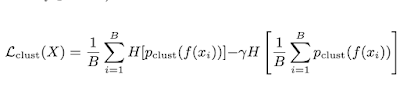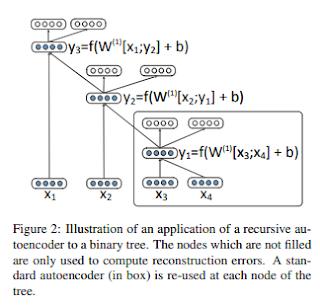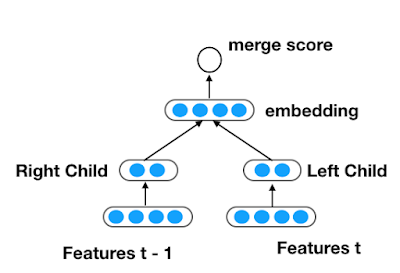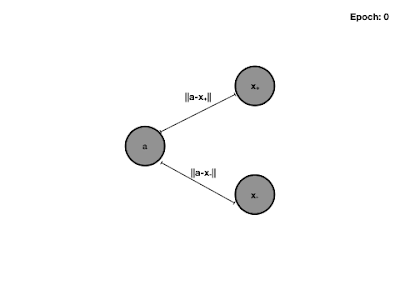## Sunday, September 27, 2020

### Implementing Clustering Loss For Deep Learning in Tensorflow 2.0

####The first term is the average entropy of the probabilities for each example using the softmax probabilities.
Each example xi in a batch is first embedded f(xi) and then pushed through the softmax. This term basically encourages assignment to a single cluster. The second term is the entropy of the average probability in each cluster over the complete batch. This is done so all clusters are used and the score discourages the assignment of all instances to the same batch. Below I posted my code for the loss function in Tensorflow.


class ClusteringLoss(Loss):

def __init__(self, gamma=1.0):
super().__init__()
self.gamma = gamma

def call(self, true, pred):
y  = pred + 1e-6
h1 = -1.0 * tf.reduce_sum(
tf.math.log(y) * y, axis=-1
)
H1 = tf.math.reduce_mean(h1)
total_y = tf.math.reduce_mean(y, axis=0)
H2 = -1 * tf.reduce_sum(
tf.math.log(total_y) * total_y
)
return H1 - self.gamma * H2



#### REFERENCES

 Jensen et. al.: "Coincidence, Categorization, and Consolidation: Learning to Recognize Sounds with Minimal Supervision", ICASSP 2020.

## Thursday, March 12, 2020

### Recursive Auto Encoders in Tensorflow 2.0

In my last post, I described recursive neural networks and how they can be used in PyTorch.
In this post, I will describe the recursive autoencoder and my implementation in TensorFlow 2.0 using the new eager execution functionality and the gradient tape.

### What is a recursive autoencoder?Figure from the paper explaining auto encoders

As with the regular autoencoder we build a neural network that decides if we merge two inputs. Instead of having a target, in the autoencoder case we simply reconstruct the input and merge the nodes with the lowest reconstruction error in a greedy manner.

### Implementation

First, we define the auto encoder:

def merge_encoder(n_in):
a = tf.keras.layers.Input(n_in)
b = tf.keras.layers.Input(n_in)
c = tf.keras.layers.Concatenate()([a,b])
h = tf.keras.layers.Dense(n_in)(c)
o = tf.keras.layers.Dense(n_in * 2)(h)
merge = tf.keras.models.Model(inputs=[a, b], outputs=[h, c, o])
return merge

The model has two inputs that should be merged. These will be concatenated and fed through a dense layer resulting in the hidden representation. The hidden representation is directly used to reconstruct the input. In order to keep track of the merges, I define a node class.

class Node:

def __init__(self, i, embedding, score, l = None, r = None):
self.i = i
self.score = score
self.embedding  = embedding
self.left = l
self.right = r

def print(self, offset=""):
print("{} {} {} {}".format(offset, self.i, self.score, np.mean(self.embeding)))
if self.left is not None and self.right is not None:
self.left.print(offset + "\t")
self.right.print(offset + "\t")

def merge(self, other, merger):
merged = merger([self.embedding, other.embedding])
h = merged
c = merged
y = merged
score = tf.nn.l2_loss(y - c) + self.score + other.score
return Node(-1, h, score, self, other)

def ts2leafs(ts):
return [Node(i,ts[i], tf.constant(0.0)) for i in range(0, len(ts))]


A merge holds the embedding, an id for all leaf nodes as well as the merge score. Merging nodes will involve computing the hidden representation for the new node and computing the score from the reconstruction error. At the beginning every vector in a sequence is simply a leaf node. Now we can greedily merge the sequences by finding the minimum possible reconstruction error. We keep merging until only one node is left.


def merge(x, m):
if len(x) > 1:
min_loss = float('inf')
min_node = None
min_i = 0
min_j = 0
for i in range(len(x)):
for j in range(len(x)):
if i < j:
node = x[i].merge(x[j], m)
if node.score < min_loss:
min_node = node
min_loss = node.score
min_i = i
min_j = j
next_x = x.copy()
next_x[min_i] = min_node
next_x = [next_x[idx] for idx in range(0, len(x)) if idx != min_j]
return merge(next_x, m)
else:
return x

Now we can learn the model using TensorFlow's gradient tape which keeps track of all computations during our merges, compute the gradient and update the model.


m = merge_encoder(10)
x = ts2leafs([np.ones((1, 10)) * i for i in range(0, 10)] + [np.ones((1, 10)) * i for i in range(0, 10)])
for i in range(0, 100):
tape.watch(m.variables)
node = merge(x, m)
print(node.score)
m.save('merger.h5')


My code can be found in this gist

## Saturday, September 28, 2019

### Recursive Neural NetworksA Recursive Neural Network

Recursively Neural Networks can learn to structure data such as text or images hierarchically. The network can be seen to work similar to a context free grammar and the output is a parse t
The figure below (from this paper) shows examples of parse trees for an image (top) and a sentence (bottom).

A recursive neural network for sequences always merges two consecutive input vectors and predicts if these two vectors can be merged. If so, we replace the two vectors with best merging score with the hidden vector responsible for the prediction. If done in a recursive manner, we can construct a parse tree.

Formally we can construct the network as follows. Given an input sequence $x = x_1 ... x_T, x_i \in \mathbb{R}^D$ then for two neighboring inputs $x_t, x_{t + 1}$ we predict a hidden representation:

$h_t = \sigma(x_t W_l + b_l)$
$h_{t + 1} = \sigma(x_{t + 1} W_r + b_r)$

The hidden layer used for replacement is computed from the concatenation of the two previous hidden layers: $h=\sigma([h_t, h_{t + 1}] W_h + b_h)$. Finally, the prediction is simply another layer predicting the merging score.

For the implementation we define the neural network with the merging decision first. Given a node with the parent representations (for leaf nodes that are the input vectors) we build the hidden representations and then compute the merging score.
class NeuralGrammar(nn.Module):
'''
Given a tree node we project the left child and right child into
a feature space cl and cr. The concatenated [cl, cr] is projected
into the representation of that node. Furthermore, we predict
the label from the hidden representation.
'''

def __init__(self):
super(NeuralGrammar, self).__init__()
self.left       = nn.Linear(IN, H, bias=True)
self.right      = nn.Linear(IN, H, bias=True)
self.parent     = nn.Linear(2 * H, IN, bias=True)
self.projection = nn.Linear(IN, 1, bias=True)

def forward(self, node):
l = node.left_child.representation
r = node.right_child.representation
y = node.label
x = torch.cat([torch.relu(self.left(l)), torch.relu(self.right(r))], 0)
p = torch.tanh(self.parent(x))
score = self.projection(p)
return (p, score)


Now we can implement our node class which also handles the parsing, by greedily merging up to a tree. A node in the tree holds it's representation as well as a left and a right child. In other words, each node represents a merging decision with the two children being the nodes merged and the parent representation being the hidden layer in the merger grammar. Parsing a tree involves merging the vectors with the highest score and replacing the nodes with their parent node.

class TreeNode:
'''
A tree node in a neural grammar.
Represented as a binary tree. Each node is also represented by
an embedding vector.
'''

def __init__(self, representation=None):
self.representation = representation
self.left_child     = None
self.right_child    = None

def greedy_tree(cls, x, grammar):
'''
Greedily merges bottom up using a feature extractor and grammar
'''
T, D   = x.shape
leafs  = [cls(x[i, :]) for i in range(T)]
while len(leafs) >= 2:
max_score       = float('-inf')
max_hypothesis  = None
max_idx         = None
for i in range(1, len(leafs)):
# difference of reconstruction of the center of the span of the subtree in the spectrogram is the score
# inspired by continuous bag of words
hypothesis = cls(leafs[i - 1].start, leafs[i].stop)
hypothesis.left_child     = leafs[i - 1]
hypothesis.right_child    = leafs[i]
representation, score     = grammar(hypothesis)
hypothesis.representation = representation
if score > max_score:
max_score      = score
max_hypothesis = hypothesis
max_idx        = i
leafs[max_idx - 1:max_idx + 1] = [max_hypothesis]
return leafs


During learning, we collect the scores of the subtrees and use labels to decide if a merge was correct or not.

## Thursday, August 1, 2019

### Code Along Books: Bitcoin, Go Game, Ray TracersA scene rendered with your own ray tracer

I like learning by doing. In my opinion the only real self test whether you understood something is a working prototype. For example, when learning more about the details of deep learning or xgboost, I implemented my own auto differentiation and gradient boosted trees.

Recently I found several books that guide you through implementations of fairly complex pieces of software. After finishing a chapter it feels like making progress on a project. So it is easier to follow along and you don't have to search for all the references needed yourself. You still have the benefit from learning by doing.

The book based projects I found are:
1. A ray tracer 
2. A go AI 
3. A bitcoin implementation 
AI in lisp and I wanted to implement my prolog in scala.

The previous projects on neural networks were based on Goodfellow's Deep Learning book  and many other references such as online classes so it was not as easy to code along. The same is true for the gradient boosting implementation which was mostly based on papers and books.

However the other projects simply involved writing code and the book was the only thing required to get into the topic. The quality all of these books show is that it is not a collection of separated code pieces that explain a topic but the topic's are explained by a coherent code based that you develop yourself form scratch.

### 1. A Ray Tracer

The book "The Ray Tracer Challenge: A Test Driven Guide to Your First 3D Rendered" , is giving you no actual code but behavior specifications (cucumber). The code has to be written by yourself.
Harder parts include pseudo code. The book covers the basic math library and then uses the defined primitives to construct more and more complex features.
The end result is a full ray tracer with shadows reflections and multiple primitives.

### 2. A Go AI

In the next book: "Deep Learning And The Game Of Go" , you build a go AI using the code provided by the authors. The books walks you through the implementation of the go board and rules first. Then the authors walk through classic game AI algorithms such as monte carlo tree search. In the end the authors introduce game state evaluation using convolutional neural networks using Keras.
The resulting system resembles an early version of AlphaGO

### 3. Bitcoin

The book "Programming Bitcoin"  is a mix between the first two. Lot's of code is provided in the form of skeletons but the reader still has to fill in the blanks for several assignments. The book
covers everything from basic cryptography to scripting bitcoin. The end result is a full bitcoin
implementation.

### Wrap up

The great thing about all the books mentioned is that all these books do cover the primitives needed too. You don't need to rely on a library but the authors walk you front to back.

My code bases are all on github. Some are work in progress [WIP] since
i did not finish the books yet. For example, in books 2 and 3 I only went through the first chapters:

### REFERENCES:

 Buck, "The Ray Tracer Challenge: A Test Driven Guide to Your First 3D Rendered", Pragmatic Bookshelf, 2019
 Pumperla, Furguson, "Deep Learning And The Game Of Go", Manning, 2019
 Song, "Programming Bitcoin: Learn How To Program Bitcoin From Scratch", Oreilly, 2019
 Norvig: "Paradigmes of Artificial Intelligence Programming: Case Studies in Lisp", Morgan Kaufmann, 1992
 Ian Goodfellow, "Deep Learning", MIT Press, 2016

## Tuesday, July 9, 2019

### WebRTC and Tensorflow.jsWebCam image classification on web page

I will give a quick tutorial on how to connect a webcam in html5/WebRTC to Tensorflow.js for image classification. We will load a pre trained mobile net and then pass it frames from the camera. First, we define our html page and specify a video element in which we later stream the video from the camera,
a label field and also start the WebRtc + neural network code. The code can be found at this gist.

<!DOCTYPE html>
<html>
<title> Hello WebRTC </title>
<script src="https://cdn.jsdelivr.net/npm/@tensorflow/tfjs@1.0.0/dist/tf.min.js"></script>
<body>
<video id="cam" width="224" height="224" autoplay playsinline></video> <br/>
<script src="camnet.js"></script><br/>
<font color="red" size="5"> LABEL: </font><br/>
<div id="label"> </div>
</body>
</html>


 async function init() {
try {
const constraints = window.constraints = {audio: false, video: true};
onSuccess(stream, net);
} catch (e) {
onError(e);
}
}


The camera stream can be retrieved by the getUserMedia function. The onError method simply writes an error to the console. If we are successful, we get the video element from our dom and bind the stream to the video. We then start the detection loop with a method called onFrame.
function onSuccess(stream, net) {
const video = document.querySelector('video');
const videoTracks = stream.getVideoTracks();
console.log('Got stream with constraints:', constraints);
console.log(Using video device: ${videoTracks.label}); window.stream = stream; video.srcObject = window.stream; onFrame(video, net); }  onFrame's inner function processFrame is an infinite recursion. We grab the video frame by frame and push it into a classify method along with the neural network, the video element as well as the label element. function onFrame(video, net) { var label_element = document.getElementById('label'); console.log(net.summary()); async function processFrame() { classify(video, label_element, net) requestAnimationFrame(processFrame); } processFrame(); }  The last method transforms the camera image into a tensor, normalizes the color and then construct a batch with only one example. Based on the prediction from the mobile net, we extract the best class and write it into the label element. async function classify(img_element, label_element, net) { const img = tf.browser.fromPixels(img_element).toFloat(); const offset = tf.scalar(127.5); const normalized = img.sub(offset).div(offset); const batched = normalized.reshape([1, IMAGE_SIZE, IMAGE_SIZE, 3]); const prediction = await net.predict(batched).data(); var max_i = 0; var max_v = prediction; for (let i = 0; i < prediction.length; i++) { if(prediction[i] > max_v) { max_v = prediction[i]; max_i = i; } } const label = IMAGENET_CLASSES[max_i]; if (max_v > 0.5) { label_element.innerHTML = label + " [" + max_v + "]"; } }  ## Sunday, July 7, 2019 ### Implementing Triplet Loss Function in Tensorflow 2.0 In this post I will go through an implementation of the triplet loss for siamese neural network architectures in keras (tensorflow 2.0). The goal of training a neural network with a triplet loss is to learn a metric embedding. That is examples that are conceptually close are also close in euclidean space and examples that are conceptually further are further away in euclidean space, too. The triplet loss is introduced in the facenet paper from google. When learning with the triplet loss, we choose three examples. The first example is called the anchor, which can be any example from our dataset. The positive example is one that is conceptually close. For example, a conceptually close example for face recognition might be a picture of the same person as shown in the anchor image. The negative example might simply be a picture of any other person. The embedding network can actually have any architecture. During training, we push all three examples through the network, compute the loss function with the three embeddings and adjust the weights which in each epoch pushes negative examples further from it's anchors and positive examples closer.Figure 1: The Triplet loss forces negative examples further from the anchor and positive examples closer. An example is shown below. The loss consists of three parts. The first is the euclidean distance between the anchor and it's positive example. The second is the euclidean distance between the anchor and it's negative example. Since we want to minimize the loss, the first term should be small and the second term should be large. Furthermore, we introduce a regularization term or margin between the examples.Figure 2: We embed the anchor, positive and negative examples with the same network and apply the triplet loss. During Backpropagation we achieve higher euclidean distances to the negative example and lower distances for the negative examples. For unsupervised audio embedding, one way to sample triplets is to pick a window from the audio as the anchor and a close window in time to the anchor as positive (since audio does not change that rapidly). The negative example is simply a sample from another file. In order to implement such a loss in Tensorflow 2.0 / Keras, we can implement the Loss base class. class TripletLoss(loss.Loss): def __init__(self, margin): super().__init__() self.margin = margin def call(self, y_true, y_pred): anchor = y_pred pos = y_pred neg = y_pred pos_dist = tf.reduce_sum(tf.square(tf.subtract(anchor, pos)), axis=-1) neg_dist = tf.reduce_sum(tf.square(tf.subtract(anchor, neg)), axis=-1) basic_loss = tf.add(tf.subtract(pos_dist, neg_dist), self.margin) loss = tf.reduce_sum(tf.maximum(basic_loss, 0.0)) return loss  As you can see from the code, we do not need the ground truth (y truth) and we simply pass dummy values during training (Keras will still check it's dimension). In order to construct a model using the triplet loss, we can build an embedder model and then use that model three times in the triplet loss model. During back propagation, the three gradients will be summed and then passed through the embedder model (deep learning book chapter 6, Algorithm 6.6). In order to see a full example for audio data, you can check this gist. ## Monday, April 1, 2019 ### Navigable Small World Graphs for Approximate Nearest Neighbors: Implementation in Rust and Time Series Experiments.A navigable small world graph. Image from NSWG A small world graph or network, is a mathematical graph with certain properties. In such a graph most nodes will not be neighbors but two neighbors of a node will likely be. In other words, traversing the graph from one node to the other can be achieved in very few edge transitions. The idea is to use such a structure to support fast approximate nearest neighbor queries. In a navigable small world graph (NSWG) each node or vertex is associated with a vector in$\mathbb{R}^d$. During search, we check the distance to all neighbors of the node and transition to the closest one. We then repeat the search until we found a local minimum. In this post we discuss how to compute the$kclosest neighbors and explore the method using the UCR time series dataset. The code can be found on github. ### Modelling the Graph and Search Results The edges in the graph are implemented using a map. For each vertex, we map to the neighboring vertices. The struct also holds the flattened vectors associated with each vertex. /// A navigable small world graph defined /// by a sparse edge set and a flat vector set, one for each vertex. pub struct NavigableSmallWorldGraph { pub edges: HashMap<usize, Vec<usize>>, pub instances: Vec<f32>, pub dim: usize }  A search result is simply implemented as a struct with a node id as well as a distance to the query. pub struct SearchResult { pub node: usize, pub distance: f32 }  Furthermore, we implement comparing to search results by their distance. In order to manage the set of candidates during knn search, we implement a result set as a bineary tree set. In this way, extracting the top neighbors is fast. pub struct ResultStore { ordered: BTreeSet<SearchResult> } impl ResultStore { pub fn new() -> ResultStore { ResultStore { ordered: BTreeSet::new() } } pub fn len(&self) -> usize { self.ordered.len() } pub fn take(&self, k: usize) -> Vec<SearchResult> { self.ordered.iter().take(k).map(|x| x.clone()).collect() } pub fn best(&self, at: usize) -> SearchResult { let k_best = self.ordered.iter().take(at); if k_best.len() < at { SearchResult::none() } else { k_best.last().unwrap().clone() } } pub fn insert(&mut self, result: SearchResult) { self.ordered.insert(result); } pub fn remove(&mut self, result: &SearchResult) { self.ordered.remove(result); } }  Insertion in this data structure automatically inserts in order. The same goes for the remove option, which keeps the tree ordered. Iterating the elements now allows to get the candidates sorted. ### KNN-Search and Graph Construction First we define the distances we support. For this post, I implement the euclidean distance between a query vector and a node in the graph as well as the dynamic time warping distance, when seeing the vectors as time series. If we give a warping band to the distance function, we compute the dtw distance.  fn distance(&self, query: &[f32], node: usize, align_band: Option) -> f32 { match align_band { Some(w) => self.dtw(query, node, w), None => self.euclidean(query, node) } } fn euclidean(&self, query: &[f32], node: usize) -> f32 { assert!(query.len() == self.dim); let y = &self.instances[node * self.dim .. (node + 1) * self.dim]; let mut distance = 0.0; for i in 0 .. query.len() { distance += f32::powf(query[i] - y[i], 2.0); } f32::sqrt(distance) } fn dtw(&self, query: &[f32], node: usize, w: usize) -> f32 { let y = &self.instances[node * self.dim .. (node + 1) * self.dim]; let n = query.len(); let m = y.len(); // allocating here is the main bottleneck let mut dp = vec![std::f32::INFINITY; (n + 1) * (m + 1)]; dp = 0.0; for i in 1 .. n + 1 { for j in usize::max(NavigableSmallWorldGraph::sub(i, w), 1) .. usize::min(i + w, m + 1) { let distance = f32::powf(query[i - 1] - y[j - 1], 2.0); dp[i * (n + 1) + j] = distance + NavigableSmallWorldGraph::min3( dp[(i - 1) * (n + 1) + j], dp[i * (n + 1) + (j - 1)], dp[(i - 1) * (n + 1) + (j - 1)] ) } } dp[dp.len() - 1] }  We can now turn to the search algorithm. During the search, we maintain an ordered candidate lost, an ordered result list and an unordered visited set. During a search run, we start with a random entry node. We then add said node as the first candidate. In the following steps, we get the best node from the candidate list and add all of it's neighbors as candidates, as long as we did not visit the node before. If a candidate is further away then the k-th neighbor so far, we stop. We restart the searchn\$ times from different random start points.
    /// Given a query find the k-nearest neighbors using the nswg graph
/// * query The query vector
/// * n_searches number of restarts
/// * k_neighbors number of results
/// * align_band Sakoe Chiba Band for Dynamic Time Warping, if not set we use Euclidean Distance
pub fn search(
&self,
query: &[f32],
n_searches: usize,
k_neighbors: usize,
align_band: Option<usize>,
) -> (Vec<SearchResult>, usize) {
let mut candidates = ResultStore::new();
let mut results = ResultStore::new();
let mut visited = HashSet::new();
let mut n_steps = 0;
for _attempt in 0..n_searches {
let mut bsf = ResultStore::new();
// stop if we visited all of the nodes
if self.n_instances() == visited.len() {
break;
}
// sample entry point, make sure we never used it before
// and insert the point as a candidate
let mut entry_point = rng.gen_range(0, self.n_instances());
while visited.contains(&entry_point) {
entry_point = rng.gen_range(0, self.n_instances());
}
let distance = self.distance(query, entry_point, align_band);
candidates.insert(SearchResult::new(entry_point, distance));
while candidates.len() > 0 {
// check if we can stop: abendon search if the result is the worst than the top k so far
let candidate = candidates.best(1);
let kth = f32::min(
results.best(k_neighbors).distance,
bsf.best(k_neighbors).distance,
);
candidates.remove(&candidate);
if candidate.distance > kth {
break;
}
for edge in self.edges[&candidate.node].iter() {
if !visited.contains(edge) {
n_steps += 1;
visited.insert(edge);
let edge = *edge;
let distance = self.distance(query, edge, align_band);
candidates.insert(SearchResult::new(edge, distance));
bsf.insert(SearchResult::new(edge, distance));
}
}
}
for res in bsf.take(k_neighbors) {
results.insert(res);
}
}
(results.take(k_neighbors), n_steps)
}


### Testing the Code on UCR Time Series

In order to test the code, we run it against multiple datasets from the UCR time series archive. Our accuracy is similar to the UCR datasets. However, the number of distance computations is way less.